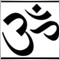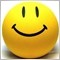# Recreating heiken ashi candles with moving averages131

Is there an easy way to recreate heiken ashi candles using moving averages? The code for heiken ashi candles themselves is a bit involved. As the calculation is basicly working out a moving average cross I wonder if anyone knows the best two ma's and settings to use?

I have an alert dashboard already coded which uses moving averages so it would be very easy just to change the ma settings to get it to do what I want. Thanks.5300

heikin_ashi. Theres another thread about HA running next to this one. Perhaps the both of you can exchange ideas. There's nothing mql4 or automated about these topics. Perhaps you can OverLay a Moving_Average on heikin_ashi and get your answers that way.131

Ok, thanks.2092

hughbriss:

Is there an easy way to recreate heiken ashi candles using moving averages? The code for heiken ashi candles themselves is a bit involved. As the calculation is basicly working out a moving average cross I wonder if anyone knows the best two ma's and settings to use?

I have an alert dashboard already coded which uses moving averages so it would be very easy just to change the ma settings to get it to do what I want. Thanks.

Open Heikin Ashi in MetaEditor, and do this

1. Do these find and add

```#property indicator_width3 3  //<--- find this
#property indicator_width4 3  //<--- find this

//----
extern int   ma_period= 5;    //<<-- add this
extern int   ma_method=MODE_SMMA; //<<-- add this
extern int   ma_shift=0;      //<<-- add this
extern color color1 = Red;    //<--- find this
extern color color2 = White;  //<--- find this```

2. Find these

```haClose=(Open[pos]+High[pos]+Low[pos]+Close[pos])/4;
haHigh=MathMax(High[pos], MathMax(haOpen, haClose));
haLow=MathMin(Low[pos], MathMin(haOpen, haClose));```

... and replace them with these

```haClose=(ma (PRICE_OPEN, pos)+ma (PRICE_HIGH, pos)+ma (PRICE_LOW, pos)+ma (PRICE_CLOSE, pos))/4;
haHigh=MathMax(ma (PRICE_HIGH, pos), MathMax(haOpen, haClose));
haLow=MathMin(ma (PRICE_LOW, pos), MathMin(haOpen, haClose));```

3. Do find and add again

```      ExtMapBuffer3[pos]=haOpen;   //<--- Find this
ExtMapBuffer4[pos]=haClose;  //<--- Find this
pos--;                  //<--- Find this
}                             //<--- Find this
//----
return(0);                      //<--- Find this
}                                //<--- Find this
//+------------------------------------------------------------------+
double ma (int price_type, int bar)                                                    //<<-- add this